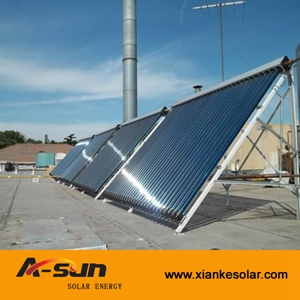Language:
News Group
How much energy can a solar collector produce?
Issue Time:2017-05-15
Using this energy calculator you may determine how much energy an solar collector will produce each day/month/year. The way you utilize this energy is up to you. You can heat water for showering and washing clothes, or central heat a building. In fact one integrated system can complete both these functions.

You can also use these values to help you calculate how much energy you can save by using an solar collector.

In order to calculate energy output you must input the following variables:

Insolation Level - Before you calculate your energy output, you must know your solar insolation level. This are available from the insolation page. Take note of your max and min levels throughout the year as well as the annual average value. When assessing potential energy savings, input annual average insolation, and take note of the "per year" energy output value.
Energy must be input in the unit kWh/m2/day. 1 kWh/m2/day = 317.1 Btu/ft2/day

Collector Size - You must enter the collector size in absorber surface area.
The absorber surface area of the various tubes sizes are as follows:
- 58/1800 = 0.08m2 per tube. Therefore an 20 tube = 1.6m2 absorber area
- 58/1500 = 0.067m2 per tube

Energy Cost - Enter cost per kWh in your local currency
(may need to convert from m3 or Therms)
1 therm = 29.3kWh = 100,000Btu = 105.5MJ
Natural Gas is 39MJ/m3 = 10.83 kWh/m3
LPG Propane (liquid) = 25.3MJ/L = 7kWh/L
LPG Propane (gas) = 93.3MJ/m3 = 25.9kWh/m3